# Plan Nuclear Fuel Disposal Using Multiobjective Optimization

This example shows how to formulate and solve a large nonlinear multiobjective problem that has some integer constraints. The problem is adapted from Montonen, Ranta, and Mäkelä . The goal is to dispose of spent nuclear fuel, with objectives of minimizing cost, minimizing the amount of time between removal of a spent nuclear fuel assembly from a reactor until it is buried, and minimizing the number of spent fuel assemblies in storage at any one time. The problem is a multiperiod planning problem, and each period is five years long.

### Model Overview

A nuclear reactor creates waste products that must be buried for long-term disposal. These waste products are fuel rod assemblies containing spent nuclear fuel. The assemblies are hot and radioactive when taken from the reactor, and gradually become less so. At period `t`, the radiation level is given by a double-exponential decay function with parameter vector `u`:

$radiation={u}_{1}\mathrm{exp}\left(-{u}_{2}t\right)+{u}_{3}\mathrm{exp}\left(-{u}_{4}t\right)$.

Each assembly remains in a pool of water in the reactor building until it is cool enough to be transferred to an interim storage facility, where it is again placed in a pool of water. When the assembly is cooled further, it can be encapsulated with other assemblies in a copper-iron canister and then buried in a disposal tunnel. All disposal tunnels connect to a central tunnel.

This figure illustrates the stages of the nuclear fuel assemblies from reactor to final disposal.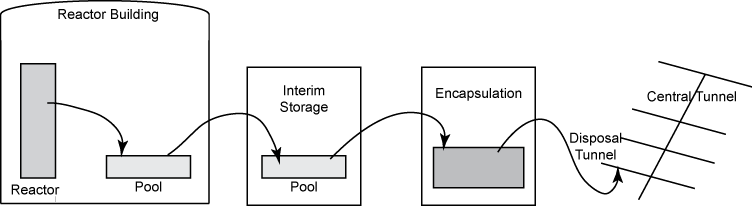The problem variables relate to a schedule where each unit of time represents 5 years. Time periods begin at 1.

### Constants Associated with Model

`Z` is the last time period in which fuel assemblies are removed from the reactor. Removal periods are `1:Z`. In , `Z` = 11. Each period is 5 years, so the last period in which fuel assemblies are removed is at time 55.

`N` is the last time period in which canisters are buried. Burial periods are `1:N`. In , `N` = 19. Each period is 5 years, so the last period in which canisters can be buried is at time 95.

```Z = 11; N = 19;```

`a` is the period of the last removal before the first disposal.

`b` is the disposal period in which the last removal occurs.

```a = 5; b = 6;```

`R` is the minimum number of periods to store an assembly.

`R = 4;`

`K` is the maximum number of assemblies to fit into one canister.

`K = 4;`

`T` is the minimum number of canisters disposed in one period.

`T = 50;`

`U` is the maximum number of canisters disposed in one period.

`U = 500;`

`Q` is the length of a disposal tunnel in meters.

`Q = 350;`

`M(i)` is the number of assemblies removed at time `i`.

`M = 300 - 60*(-1).^(1:Z); % 360 for odd indices, 240 for even`

`A(i,j)` is the storage time of an assembly from removal `i` in period `j`, where `i <= Z` and `j <= N`.

```A = zeros(Z,N); for i = 1:Z for j = i:N A(i, j) = j - i; end end```

`p(i,j)` is the decay heat power of an assembly (in watts) from removal `i` in period `j`, where `i <= Z` and `j <= N`.

```% The following parameters fit P_{i,j} of Table A2 from  to within 1 in each % entry (fractional error <= 1/250). u = [503 0.1346 260 0.0231]; myfun = @(d)round(u(1)*exp(-u(2)*d) + u(3)*exp(-u(4)*d)); PP = myfun(1:N); pij = zeros(Z,N); for i = 2:Z for j = 1:i pij(i,j) = 1e3; % Dummy values because j >= i does not occur. end end for i=1:Z pij(i,i:end) = PP(1:(N-i+1)); % Same decay profile for all removal times end```

`pmaxup` is the upper bound on the average power of a canister, and `pmaxlow` is the lower bound.

```pmaxup = 1830; pmaxlow = 1300;```

`dDTup` is the upper bound on the distance between disposal tunnels, and `dDTlow` is the lower bound.

```dDTup = 50; dDTlow = 25;```

`dCAup` is the upper bound on the distance between canisters in a disposal tunnel, and `dCAlow` is the lower bound.

```dCAup = 15; dCAlow = 6;```

The costs associated with the operations are not given in . This example assumes the following values:

• `Cas` is the storage cost for one assembly per period.

• `Cis` is the storage cost for one assembly per period in interim storage.

• `Csp` is the cost of a storage place per assembly.

• `Cca` is the cost of a canister.

• `Cef` is the cost of operating the encapsulation facility per period.

• `Cdt` is the cost of a disposal tunnel per meter.

• `Cct` is the cost of the central tunnel per meter.

```Cas = 50; Cis = 60; Csp = 10; Cca = 1200; Cef = 300; Cdt = 3000; Cct = 5000;```

### Optimization Variables for Problem

To create the problem for MATLAB®, use the problem-based approach. Define continuous variables for most quantities.

This figure illustrates the variables associated with the movement of the assemblies.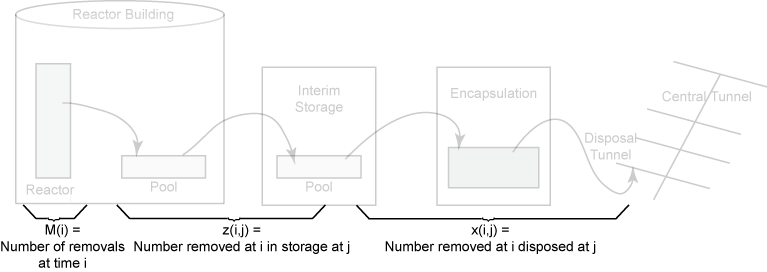`x(i,j)` is the number of assemblies removed at time `i` and disposed at time `j`, `i <= Z` and `j <= N`.

`x = optimvar("x",Z,N,LowerBound=0,UpperBound=U*K);`

`z(i,j)` is the number of assemblies removed at time `i` and in storage at time `j`, `i <= Z` and `j <= N`.

`z = optimvar("z",Z,N,LowerBound=0,UpperBound=U*K*N/2);`

`y(j)` is the number of canisters disposed at time `j <= N`.

`y = optimvar("y",N,LowerBound=0,UpperBound=U);`

`pmax` is the maximum average power of a canister.

`pmax = optimvar("pmax",LowerBound=pmaxlow,UpperBound=pmaxup);`

This figure illustrates quantities associated with the disposal tunnels.`dDT` is the distance between adjacent disposal tunnels.

`dDT = optimvar("dDT",LowerBound=dDTlow,UpperBound=dDTup);`

`dCA` is the distance between adjacent canisters in a disposal tunnel. You compute this distance later on, in the Problem Constraints section of this example, using the function `g`.

This figure relates to the encapsulation times.Specify the following variables as integer type binary variables, which have lower bounds of 0 and upper bounds of 1.

`ej(j)` indicates that the encapsulation facility is in operation during the period `j <= N`.

`ej = optimvar("ej",N,Type="integer",LowerBound=0,UpperBound=1);`

`ejON(j)` indicates that encapsulation starts at the beginning of period `j <= N`.

`ejON = optimvar("ejON",N,Type="integer",LowerBound=0,UpperBound=1);`

`ejOFF(j)` indicates that encapsulation ends at the beginning of period `j <= N`.

`ejOFF = optimvar("ejOFF",N,Type="integer",LowerBound=0,UpperBound=1);`

This figure relates to the times when assemblies can be disposed, `rij = 1`. These times start when `ejON = 1` and end when `sij = 1`.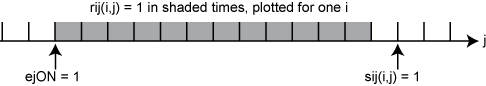`sij(i,j)` indicates that assemblies removed at time `i` can no longer be disposed starting at the beginning of time `j`, `i <= Z` and `j <= N`.

`sij = optimvar("sij",Z,N,Type="integer",LowerBound=0,UpperBound=1);`

`rij(i,j)` indicates that assemblies removed at time `i` can be disposed at time `j`, `i <= Z` and `j <= N`.

`rij = optimvar("rij",Z,N,Type="integer",LowerBound=0,UpperBound=1);`

All optimization variables and problem parameters are now defined.

### Problem Constraints

Create an optimization problem to hold the objective and constraints.

`prob = optimproblem;`

The constraint numbers match the equations in . The first three constraints relate to the number of assemblies in interim storage.

```jnot1 = 2:N; prob.Constraints.cons10 = z(:,1) - M(:) + x(:,1) == 0; prob.Constraints.cons11 = z(:,jnot1) - z(:,(jnot1 - 1)) + x(:,jnot1) == 0; prob.Constraints.cons12 = z(:,N) == 0;```

Set the constraint that all assemblies are disposed once.

`prob.Constraints.cons13 = sum(sij,2) == 1;`

Define the variable `rij` by setting the following constraints.

```cons15 = optimconstr(Z,N); cons15(:,1) = rij(:,1) == -sij(:,1) + ejON(1); % equation 14 cons15(:,jnot1) = rij(:,jnot1) == ... rij(:,jnot1-1) - sij(:,jnot1) + repmat(ejON(jnot1)',Z,1); % equation 15 prob.Constraints.cons15 = cons15;```

Set the constraint that disposal occurs only during times when the encapsulation facility is in operation.

```cons16 = rij <= repmat(ej', Z, 1); prob.Constraints.cons16 = cons16;```

Specify the constraint that production capacity is not exceeded.

`prob.Constraints.cons17 = x <= U*K*rij;`

The next constraint enforces that assemblies are cool enough before disposal.

`prob.Constraints.cons18 = x.*(A - R) >= 0;`

The following constraints relate to the encapsulation facility. These constraints enforce that the facility is turned on and off only once, which means that all canisters are encapsulated in one run,

```prob.Constraints.cons19 = sum(ejON) == 1; prob.Constraints.cons20 = sum(ejOFF) == 1;```

Define variable `ej` by setting the following constraints.

```cons21 = optimconstr(N); cons21(1) = ej(1) == ejON(1) - ejOFF(1); % equation 21 cons21(jnot1) = ej(jnot1) == ... ej(jnot1 - 1) + ejON(jnot1) - ejOFF(jnot1); % equation 22 prob.Constraints.cons21 = cons21;```

Set constraints that the number of canisters is sufficient for disposal, does not exceed production capacity, and obeys the minimum production constraint.

```prob.Constraints.cons23 = y' >= (1/K)*sum(x,1); prob.Constraints.cons24 = y <= U*ej; jnotN = 1:(N-1); prob.Constraints.cons25 = y(jnotN) >= T*(ej(jnotN) - ejOFF(jnotN + 1));```

Regarding the disposal facility, set the constraint that the heat power of canisters is allowable.

`prob.Constraints.cons26 = sum(pij.*x,1) <= pmax*y';`

Specify a nonlinear constraint on the distance between buried canisters. The function is piecewise linear, and is defined using the `max` function, which is not a supported operation for optimization expressions. Therefore, use `fcn2optimexpr` to place the constraint into `prob`.

```g = @(pmax,dDT)max([-2.26911*dDT + 0.00675*pmax + 54.5288,... -0.05833*dDT + 0.00596*pmax - 0.727083,... -0.14*dDT + 0.17701*pmax - 350.651]); dCA = fcn2optimexpr(@(pmax,dDT)g(pmax,dDT),pmax,dDT); prob.Constraints.cons29a = dCA >= dCAlow; prob.Constraints.cons29b = dCA <= dCAup;```

### Cost Objective

The first objective for this multiobjective problem is the cost, which has seven components.

`cost = optimexpr(7,1);`

1. Storage cost of assemblies. This cost is the sum of the cost per unit time multiplied by the length of time each assembly is stored.

`cost(1) = Cas*sum(A.*x,"all");`

2. Cost of interim storage. This cost is `j*ejOFF(j)` for the one component of `ejOFF` that is `1`.

`cost(2) = Cis*max(ejOFF(1)–1,2*ejOFF(2)–1,3*ejOFF(3)–1,...,N*ejOFF(N)–1)`.

To represent this expression briefly, represent `cost(2) = Cis*u` for a new optimization variable `u`, along with the constraint

`ucons = u >= ((1:N)'.*ejOFF) – 1`.

```u = optimvar("u",LowerBound=0); cost(2) = Cis*u; ucons = u >= ((1:N)'.*ejOFF) - 1; prob.Constraints.ucons = ucons;```

3. Cost of positions for assembly storage. `cost(3)` can be represented by `Csp*v1`, where `v1` is a new optimization variable, along with the constraints

`v1 >= sum(M)`

`v1 >= sum_{i=1}^j z(i,j)` for each `1 <= j <= N`

`v1 >= sum_{i=1}^Z z(i,j)` for each `b <= j <= N`.

Create these costs and associated constraints.

```v1 = optimvar("v1",LowerBound=0); cost(3) = Csp*v1; % Include the three v1 constraints given below. v1consa = v1 >= sum(M); bmin1 = 1:(b-1); v1consb = optimconstr(b-1); for j=bmin1 v1consb(j) = sum(z(1:a+j,j)) <= v1; end ell = b:N; v1consc = sum(z(:,ell),1) <= v1; prob.Constraints.v1consa = v1consa; prob.Constraints.v1consb = v1consb; prob.Constraints.v1consc = v1consc;```

4. Cost of canisters. This cost is the cost per canister times the total number of canisters buried.

`cost(4) = Cca*sum(y);`

5. Cost of running the encapsulation facility. This cost is the cost per unit time multiplied by the length of time the facility operates.

`cost(5) = Cef*sum(ej);`

6. Cost of disposal tunnels. This is the cost per unit length times the length between canisters times the total number of canisters buried.

`cost(6) = Cdt*dCA*sum(y);`

7. Cost of central tunnel. This cost is the cost per unit length times the required length of the central tunnel. The number of canisters that can be buried in one disposal tunnel is its length `Q` divided by the distance between canisters `dCA`. The length of the central tunnel is proportional to the number of buried canisters `sum(y)` and inversely proportional to `Q/dCA`, and has cost proportional to `Cct`.

`cost(7) = Cct/Q*dDT*dCA*sum(y);`

The total cost is the sum of the seven cost components. To change the scale of the total cost to match that of the other objectives, take the logarithm of the sum.

`prob.Objective.cost = log(sum(cost));`

### Safety Objectives

The problem has two objectives related to safety. Objective 2, named `safety1`, tries to minimize the maximum storage time over all removals. Objective 3, named `safety2`, tries to stop the disposal as early as possible. Define these two objectives using the helper functions `max1` and `max2`, which appear at the end of this script.

```prob.Objective.safety1 = fcn2optimexpr(@max1,A,sij); % Minimize maximum storage time, objective (2) in  prob.Objective.safety2 = fcn2optimexpr(@max2,ejOFF); % Stop disposal as early as possible, objective (5) in ```

### Set Options

Set the options for a Pareto plot as the solver proceeds. Because the problem has over 900 variables, set options to use a population size of 500, which is larger than the default. Also, because the problem contains binary variables, use the `mutationgaussian` mutation function. This mutation function works better than the default `mutationpower` for binary variables.

```opts = optimoptions("gamultiobj",PlotFcn="gaplotpareto",PopulationSize=5e2,... MutationFcn=@mutationgaussian);```

### Run Problem

The problem formulation is complete, and the options are set for this multiobjective problem. Run the problem.

```rng default % For reproducibility [sol,fval,exitflag,output] = solve(prob,Options=opts);```
```Solving problem using gamultiobj. gamultiobj stopped because the average change in the spread of Pareto solutions is less than options.FunctionTolerance. ```
```xlabel("Cost") ylabel("Safety 1") zlabel("Safety 2")```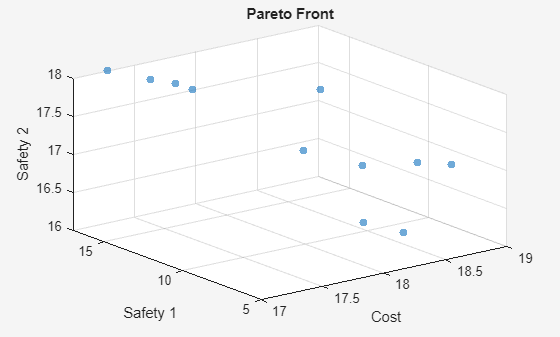The Pareto plot shows a clear tradeoff between Cost and Safety 1. The true cost is the exponential of the amount shown, so the tradeoff is more severe than illustrated.

### Examine Solution

`gamultiobj` finds several feasible solutions with varying fitness function values. To find the control variables associated with the solutions, use the data tips.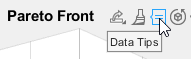After activating Data Tips, click the upper-left solution.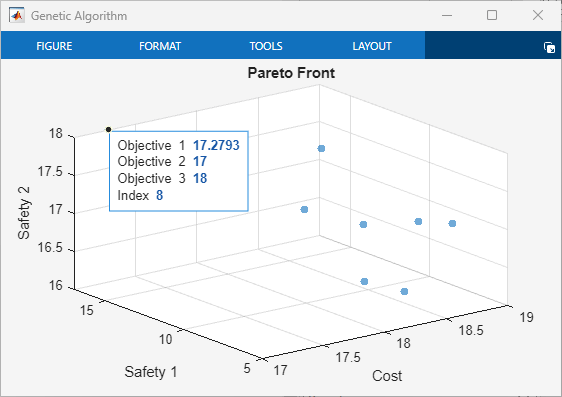The index of the selected point is 4. The variables associated with this point are in `sol(4)`.

Examine the `x` variables associated with this solution. Recall that `x(i,j)` are the removals at time `i` that are disposed at time `j`.

`disp(sol(4).x)`
``` 0 0 0 0 0 0 0 0 0 0 0 0 0 0 0 0 360.0000 0 0 0 0 0 0 0 0 0 0 0 0 0 0 0 0 0 0 240.0000 0 0 0 0 0 0 0 0 0 0 0 0 0 0 0 0 0 0 265.4535 94.5465 0 0 0 0 0 0 0 0 0 0 0 0 0 0 0 0 0 240.0000 0 0 0 0 0 0 0 0 0 0 0 0 0 0 0 0 0 0 0 360.0000 0 0 0 0 0 0 0 0 0 0 0 0 0 0 0 0 0 0 240.0000 0 0 0 0 0 0 0 0 0 0 0 0 0 0 0 0 0 254.5465 105.4535 0 0 0 0 0 0 0 0 0 0 0 0 0 0 0 0 0 0 240.0000 0 0 0 0 0 0 0 0 0 0 0 0 0 0 0 0 0 0 360.0000 0 0 0 0 0 0 0 0 0 0 0 0 0 0 0 0 0 0 240.0000 0 0 0 0 0 0 0 0 0 0 0 0 0 0 0 0 0 0 360.0000 0 ```

Clearly, the `x` schedule is not restricted to integer values. View the sums of the `x` schedule over disposals compared to the removal quantities `M(:)`.

`disp([sum(sol(4).x,2),M(:)])`
``` 360 360 240 240 360 360 240 240 360 360 240 240 360 360 240 240 360 360 240 240 360 360 ```

The `x` schedule accounts for all removals.

What are the times when the encapsulation facility is in operation?

`disp(sol(4).ej')`
``` 0 0 0 0 0 0 0 0 0 0 0 0 0 0 0 0 1 1 0 ```

The encapsulation runs for times 17 and 18.

What is the distance between disposal tunnels?

`disp(sol(4).dDT)`
``` 27.6483 ```

The distance is greater than but close to its lower bound of 25.

What is the dollar cost of the schedule? To find out, calculate `exp(sol(4).cost)`, because `sol(4).cost` is the logarithm of the total disposal cost.

`disp(exp(sol(4).cost))`
``` 2.0883e+07 ```

The cost is about \$21 million.

Examine the point in the Pareto set with the lowest value of Objective 2.The index of this point is 3. The monetary cost of this operating point is much higher.

`disp(exp(sol(3).cost))`
``` 1.4001e+08 ```

The monetary cost is about \$140 million, which is over six times the previous value. But the gain is that Objective 2 is 10 instead of 17, which corresponds to waste burial that is 35 (=7*5) years earlier. Earlier burial can be considered safer.

View the schedule of `x` for this solution.

`disp(sol(3).x)`
``` 0 0 0 0 0 0 0 0 360 0 0 0 0 0 0 0 0 0 0 0 0 0 0 0 240 0 0 0 0 0 0 0 0 0 0 0 0 0 0 0 0 0 0 0 0 0 0 160 0 200 0 0 0 0 0 0 0 0 0 0 0 0 0 0 0 0 0 240 0 0 0 0 0 0 0 0 0 0 0 0 0 0 0 0 360 0 0 0 0 0 0 0 0 0 0 0 0 0 0 0 0 0 0 0 0 240 0 0 0 0 0 0 0 0 0 0 0 0 0 0 0 0 0 0 360 0 0 0 0 0 0 0 0 0 0 0 0 0 0 0 0 0 0 0 0 0 0 240 0 0 0 0 0 0 0 0 0 0 0 0 0 0 0 0 0 360 0 0 0 0 0 0 0 0 0 0 0 0 0 0 0 0 0 0 0 0 0 0 240 0 0 0 0 0 0 0 0 0 0 0 0 0 0 0 360 0 0 0 0 ```

View the sums of the `x` schedule over disposals compared to the removal quantities `M(:)`.

`disp([sum(sol(3).x,2),M(:)])`
``` 360 360 240 240 360 360 240 240 360 360 240 240 360 360 240 240 360 360 240 240 360 360 ```

Again, the schedule accounts for all removals.

What are the times when the encapsulation facility is in operation?

`disp(sol(3).ej')`
``` 1 1 1 1 1 1 1 1 1 1 1 1 1 1 1 1 1 1 0 ```

The encapsulation runs for times 1 through 18.

What is the distance between disposal tunnels for this solution?

`disp(sol(3).dDT)`
``` 38.4942 ```

This time, the distance between disposal tunnels is about halfway between the lower bound of 25 meters and upper bound of 50 meters.

### Conclusion

This example shows the formulation of a nonlinear, multiobjective, mixed-integer optimization problem using the problem-based approach. The Data Tips in the Pareto plot enable you to analyze the solution. Instead of specifying the `gaplotpareto` plot function, you can use the `paretoplot` function to obtain a similar plot from the solution.

### References

 Montonen, Outi, Timo Ranta, and Marko M. Mäkelä. Planning the Schedule for the Disposal of the Spent Nuclear Fuel with Interactive Multiobjective Optimization. Algorithms Vol. 12, Issue 12, 2019. Available at `https://www.mdpi.com/1999-4893/12/12/252`.

### Helper Functions

This code creates the `max1` helper function.

```function v = max1(A,sij) v = round(max(max(A.*sij - 1))); % "round" ensures integer values end```

This code creates the `max2` helper function.

```function v = max2(ejOFF) v = round(max((ejOFF').*(1:length(ejOFF)) - 1)); end```